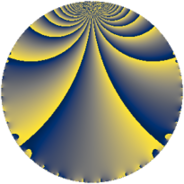# Properties

 Label 320.2.c.aLevel $320$ Weight $2$ Character orbit 320.c Analytic conductor $2.555$ Analytic rank $0$ Dimension $2$ CM discriminant -4 Inner twists $4$

# Related objects

## Newspace parameters

 Level: $$N$$ $$=$$ $$320 = 2^{6} \cdot 5$$ Weight: $$k$$ $$=$$ $$2$$ Character orbit: $$[\chi]$$ $$=$$ 320.c (of order $$2$$, degree $$1$$, not minimal)

## Newform invariants

 Self dual: no Analytic conductor: $$2.55521286468$$ Analytic rank: $$0$$ Dimension: $$2$$ Coefficient field: $$\Q(\sqrt{-1})$$ Defining polynomial: $$x^{2} + 1$$ Coefficient ring: $$\Z[a_1, \ldots, a_{5}]$$ Coefficient ring index: $$2$$ Twist minimal: no (minimal twist has level 160) Sato-Tate group: $\mathrm{U}(1)[D_{2}]$

## $q$-expansion

Coefficients of the $$q$$-expansion are expressed in terms of $$i = \sqrt{-1}$$. We also show the integral $$q$$-expansion of the trace form.

 $$f(q)$$ $$=$$ $$q + ( -1 + 2 i ) q^{5} + 3 q^{9} +O(q^{10})$$ $$q + ( -1 + 2 i ) q^{5} + 3 q^{9} + 4 i q^{13} + 8 i q^{17} + ( -3 - 4 i ) q^{25} + 10 q^{29} -12 i q^{37} -10 q^{41} + ( -3 + 6 i ) q^{45} + 7 q^{49} -4 i q^{53} -10 q^{61} + ( -8 - 4 i ) q^{65} -16 i q^{73} + 9 q^{81} + ( -16 - 8 i ) q^{85} + 10 q^{89} -8 i q^{97} +O(q^{100})$$ $$\operatorname{Tr}(f)(q)$$ $$=$$ $$2q - 2q^{5} + 6q^{9} + O(q^{10})$$ $$2q - 2q^{5} + 6q^{9} - 6q^{25} + 20q^{29} - 20q^{41} - 6q^{45} + 14q^{49} - 20q^{61} - 16q^{65} + 18q^{81} - 32q^{85} + 20q^{89} + O(q^{100})$$

## Character values

We give the values of $$\chi$$ on generators for $$\left(\mathbb{Z}/320\mathbb{Z}\right)^\times$$.

 $$n$$ $$191$$ $$257$$ $$261$$ $$\chi(n)$$ $$1$$ $$-1$$ $$1$$

## Embeddings

For each embedding $$\iota_m$$ of the coefficient field, the values $$\iota_m(a_n)$$ are shown below.

For more information on an embedded modular form you can click on its label.

Label $$\iota_m(\nu)$$ $$a_{2}$$ $$a_{3}$$ $$a_{4}$$ $$a_{5}$$ $$a_{6}$$ $$a_{7}$$ $$a_{8}$$ $$a_{9}$$ $$a_{10}$$
129.1
 − 1.00000i 1.00000i
0 0 0 −1.00000 2.00000i 0 0 0 3.00000 0
129.2 0 0 0 −1.00000 + 2.00000i 0 0 0 3.00000 0
 $$n$$: e.g. 2-40 or 990-1000 Significant digits: Format: Complex embeddings Normalized embeddings Satake parameters Satake angles

## Inner twists

Char Parity Ord Mult Type
1.a even 1 1 trivial
4.b odd 2 1 CM by $$\Q(\sqrt{-1})$$
5.b even 2 1 inner
20.d odd 2 1 inner

## Twists

By twisting character orbit
Char Parity Ord Mult Type Twist Min Dim
1.a even 1 1 trivial 320.2.c.a 2
3.b odd 2 1 2880.2.f.n 2
4.b odd 2 1 CM 320.2.c.a 2
5.b even 2 1 inner 320.2.c.a 2
5.c odd 4 1 1600.2.a.l 1
5.c odd 4 1 1600.2.a.m 1
8.b even 2 1 160.2.c.a 2
8.d odd 2 1 160.2.c.a 2
12.b even 2 1 2880.2.f.n 2
15.d odd 2 1 2880.2.f.n 2
16.e even 4 1 1280.2.f.c 2
16.e even 4 1 1280.2.f.d 2
16.f odd 4 1 1280.2.f.c 2
16.f odd 4 1 1280.2.f.d 2
20.d odd 2 1 inner 320.2.c.a 2
20.e even 4 1 1600.2.a.l 1
20.e even 4 1 1600.2.a.m 1
24.f even 2 1 1440.2.f.c 2
24.h odd 2 1 1440.2.f.c 2
40.e odd 2 1 160.2.c.a 2
40.f even 2 1 160.2.c.a 2
40.i odd 4 1 800.2.a.e 1
40.i odd 4 1 800.2.a.f 1
40.k even 4 1 800.2.a.e 1
40.k even 4 1 800.2.a.f 1
60.h even 2 1 2880.2.f.n 2
80.k odd 4 1 1280.2.f.c 2
80.k odd 4 1 1280.2.f.d 2
80.q even 4 1 1280.2.f.c 2
80.q even 4 1 1280.2.f.d 2
120.i odd 2 1 1440.2.f.c 2
120.m even 2 1 1440.2.f.c 2
120.q odd 4 1 7200.2.a.y 1
120.q odd 4 1 7200.2.a.bb 1
120.w even 4 1 7200.2.a.y 1
120.w even 4 1 7200.2.a.bb 1

By twisted newform orbit
Twist Min Dim Char Parity Ord Mult Type
160.2.c.a 2 8.b even 2 1
160.2.c.a 2 8.d odd 2 1
160.2.c.a 2 40.e odd 2 1
160.2.c.a 2 40.f even 2 1
320.2.c.a 2 1.a even 1 1 trivial
320.2.c.a 2 4.b odd 2 1 CM
320.2.c.a 2 5.b even 2 1 inner
320.2.c.a 2 20.d odd 2 1 inner
800.2.a.e 1 40.i odd 4 1
800.2.a.e 1 40.k even 4 1
800.2.a.f 1 40.i odd 4 1
800.2.a.f 1 40.k even 4 1
1280.2.f.c 2 16.e even 4 1
1280.2.f.c 2 16.f odd 4 1
1280.2.f.c 2 80.k odd 4 1
1280.2.f.c 2 80.q even 4 1
1280.2.f.d 2 16.e even 4 1
1280.2.f.d 2 16.f odd 4 1
1280.2.f.d 2 80.k odd 4 1
1280.2.f.d 2 80.q even 4 1
1440.2.f.c 2 24.f even 2 1
1440.2.f.c 2 24.h odd 2 1
1440.2.f.c 2 120.i odd 2 1
1440.2.f.c 2 120.m even 2 1
1600.2.a.l 1 5.c odd 4 1
1600.2.a.l 1 20.e even 4 1
1600.2.a.m 1 5.c odd 4 1
1600.2.a.m 1 20.e even 4 1
2880.2.f.n 2 3.b odd 2 1
2880.2.f.n 2 12.b even 2 1
2880.2.f.n 2 15.d odd 2 1
2880.2.f.n 2 60.h even 2 1
7200.2.a.y 1 120.q odd 4 1
7200.2.a.y 1 120.w even 4 1
7200.2.a.bb 1 120.q odd 4 1
7200.2.a.bb 1 120.w even 4 1

## Hecke kernels

This newform subspace can be constructed as the intersection of the kernels of the following linear operators acting on $$S_{2}^{\mathrm{new}}(320, [\chi])$$:

 $$T_{3}$$ $$T_{11}$$

## Hecke characteristic polynomials

$p$ $F_p(T)$
$2$ $$T^{2}$$
$3$ $$T^{2}$$
$5$ $$5 + 2 T + T^{2}$$
$7$ $$T^{2}$$
$11$ $$T^{2}$$
$13$ $$16 + T^{2}$$
$17$ $$64 + T^{2}$$
$19$ $$T^{2}$$
$23$ $$T^{2}$$
$29$ $$( -10 + T )^{2}$$
$31$ $$T^{2}$$
$37$ $$144 + T^{2}$$
$41$ $$( 10 + T )^{2}$$
$43$ $$T^{2}$$
$47$ $$T^{2}$$
$53$ $$16 + T^{2}$$
$59$ $$T^{2}$$
$61$ $$( 10 + T )^{2}$$
$67$ $$T^{2}$$
$71$ $$T^{2}$$
$73$ $$256 + T^{2}$$
$79$ $$T^{2}$$
$83$ $$T^{2}$$
$89$ $$( -10 + T )^{2}$$
$97$ $$64 + T^{2}$$